### Dictionary Rank of a Word | Permutations & Combinations

PERMUTATIONS & COMBINATIONS Rank of the word or Dictionary order of the English words like COMPUTER, COLLEGE, SUCCESS, SOCCER, RAIN, FATHER, etc. Dictionary Rank of a Word Method of finding the Rank (Dictionary Order) of the word  “R A I N” Given word: R A I N Total letters = 4 Letters in alphabetical order: A, I, N, R No. of words formed starting with A = 3! = 6 No. of words formed starting with I = 3! = 6 No. of words formed starting with N = 3! = 6 After N there is R which is required R ----- Required A ---- Required I ---- Required N ---- Required RAIN ----- 1 word   RANK OF THE WORD “R A I N” A….. = 3! = 6 I……. = 3! = 6 N….. = 3! = 6 R…A…I…N = 1 word 6 6 6 1 TOTAL 19 Rank of “R A I N” is 19 Method of finding the Rank (Dictionary Order) of the word  “F A T H E R” Given word is :  "F A T H E R" In alphabetical order: A, E, F, H, R, T Words beginni

### Lesson Plan Maths Class 10 | For Mathematics Teacher

E-LESSON PLANNING  FOR MATHEMATICS TEACHER CLASS 10TH

lesson plan for maths class X cbse, lesson plans for mathematics teachers,  Method to write lesson plan for maths class 10, lesson plan for maths class X, lesson plan for mathematics grade X, lesson plan for maths teacher in B.Ed.

LESSON PLAN (Mathematics) : CLASS 10th

Techniques of Making E-Lesson Plan : Click Here

## Chapter 1 : Number System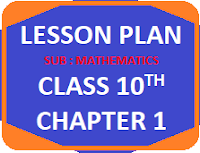This lesson plan is for the teachers who are teaching mathematics class 10th

New Lesson Plan with Technology Integration as suggested by CBSE in March, 2021
Class 10 Chapter 1 : Number System

## Chapter 2 : POLYNOMIALS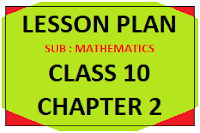This lesson plan is for the teachers who are teaching mathematics class 10th

## Chapter 3 PAIR OF LINEAR EQUATIONS IN  TWO VARIABLESThis lesson plan is for the teachers who are teaching mathematics class 10th

## Chapter 4 QUADRATIC EQUATIONS

This lesson plan is for the teachers who are teaching mathematics class 10th

## Chapter 5ARITHMATIC PROGRESSION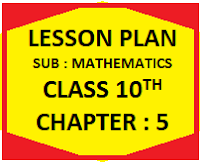This lesson plan is for the teachers who are teaching mathematics class 10th

## Chapter 6SIMILARITY OF TRIANGLESThis lesson plan is for the teachers who are teaching mathematics class 10th

## Chapter 7CO-ORDINATE GEOMETRYThis lesson plan is for the teachers who are teaching mathematics class 10th

## Chapter 8 and 9TRIGONOMETRYThis lesson plan is for the teachers who are teaching mathematics class 10th

## Chapter 10TANGENT TO THE CIRCLE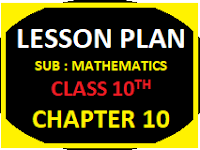This lesson plan is for the teachers who are teaching mathematics class 10th

## Chapter 11CONSTRUCTIONSThis lesson plan is for the teachers who are teaching mathematics class 10th

## Chapter 12AREA RELATED TO THE PLANE FIGURESThis lesson plan is for the teachers who are teaching mathematics class 10th

## Chapter 13SURFACE AREA & VOLUMESThis lesson plan is for the teachers who are teaching mathematics class 10th

## Chapter 14 :STATISTICS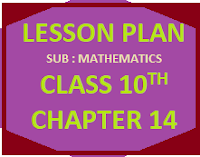This lesson plan is for the teachers who are teaching mathematics class 10th

## Chapter 15 : PROBABILITIESThis lesson plan is for the teachers who are teaching mathematics class 10th

🙏

1.This is helpful for teachers.

2.Construction chapter lesson plan link's is not working